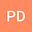Detecting Exoplanets in Multiplanetary Systems using HI Line Analysis through Gaussian Fitting
••Pritam DuttaArmy Public School, West Bengal , India, ACIC MIET, Meerut Institute Of Engineering Technology, Meerut, UP,IndiaThe paper describes the detection of exoplanets in multiplanetary systems using HI line data is an approach in astronomy. Traditional methods for detecting exoplanets have limitations in terms of sensitivity and range, which makes it difficult to detect small and distant planets. We propose a mathematical model based on the analysis of the HI line emission and absorption spectra to predict the presence of exoplanets.The model is based on fitting the observed HI line profile to a Gaussian distribution$$f(v)=Aexp[-(v-v\theta)^{2}/(2\delta v^{2})]+\delta f(v)$$ where $$\delta f(v)$$ is the perturbation caused by the exoplanet. The amplitude of the perturbation depends on the mass, orbital distance, and other properties of the exoplanet. and searching for significant deviations that may indicate the presence of an exoplanet.The chi-squared statistic,$$x^{2}$$, measures the difference between the observed and expected HI line profiles: $$x^{2}=\sum_{n=1}^{\infty}2^{-n}=1[f_{obs}(v)-f_{exp}(v)]^{2}/\sigma^{2}$$. The deviation caused by the exoplanet can be quantified using a perturbation term in the Gaussian distribution. The amplitude of the perturbation depends on the mass, orbital distance, and other properties. We use statistical tests such as the chi-squared test to measure the significance of the deviation and estimate the properties of the exoplanet and the Extragalactic distance scale.